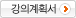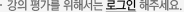### 주메뉴

### 선형계통론

• 경북대학교
• 허성호
• 주제분류
공학 >전기ㆍ전자 >전자공학
• 강의학기
2019년 2학기
• 조회수
1,244
•
강의계획서The objective of this course is to provide the students with the basics of linear systems theory and modern control engineering.

The topics covered include linear algebra, state-space representations, stability analysis, controllability/observability, and state feedback control and estimations.

We will also investigate the application of state-space methods and state feedback control to various engineering systems.

#### 차시별 강의1.1강. Introduction & Mathematical Models of Systems 1. Lecture 1_1 : Introduction- Professor Career- Overview of Control & Linear Systems- Notice to Students- Assignments and Grading Criteria1강. Introduction & Mathematical Models of Systems 2. Lecture 1_2 : Differential Equations of Physical Systems- Differential Equation of Spring Mass Damper - Electrical RLC Circuit- Kirchoff’s Current Law2.2강. Mathematical Models of Systems 1. Lecture 2_1 : Linear Approximations of Physical Systems- Characteristics of Linear Systems- The Taylor Series Expansion about the Operating Point - Second-order LTI Differential equation- Laplace Transform & Inverse Laplace Transform- Second Order Differential Equation2강. Mathematical Models of Systems 2. Lecture 2_2 : The Transfer Function of Linear Systems- The Transfer Function of Linear Systems- Block Diagram Models- Summary3.3강. Mathematical Descriptions of Systems 1. Lecture 3_1: state Space Form- Advantages of the state-space form- The state-space approach 2. Lecture 3_2: Time-domain analysis- Lack of Time domain Analysis- The frequency-domain techniques 3. Lecture 3_3: An engineering system- Dynamic, Causal,Finite-dimensional, Continuous-time- Linear Systems- Linear Time-Invariant (LTI) Systems- State Space Representation- Linearisation4.4강. Mathematical Descriptions of Systems & Linear Algebra 1. Lecture 4_1: Mathematical Descriptions of Systems Examples- Orbital Movement of a Satellite- An RLC Circuit- Discrete-time feedback system- Review and Summary 2. Lecture 4_2: Linear Algebra- Introduction- Basis- Theorem- Summary5.5강. State-Space Solutions and Realisations 1. Lecture 5_1: Solution of LTI State-Space Equations- State-Space Equation Solution of Continuous-time systems- State-Space Equation Solution of Discrete-time systems- Examples- Equivalent state equations 2. Lecture 5_2: Realisations- Controllable canonical form- Observable canonical form- Minimal realisation- SIMO, MIMO, and Multivariable System- Examples6.6강. Stability 1. Introduction- Notion of Stability- Example: Unstable System- Classification of Stability 2. Input-Output Stability of LTI Systems- Theorem of BIBO Stability- BIBO Stability of State Equations 3. Discrete-Time Case- BIBO Stability of Discrete-Time Systems 4. Internal Stability- Definition of Internal Stability 5. Lyapunov Theorem- Positive (Semi-) Definite Matrix- Lyapunov Equation7.7강. Controllability and Observability 1. Introduction 2. Controllability- Controllability and Algebraic Equivalence- Controllability Gramian 3. Observability- Luenberger Observer- Analysis with the output solution- Observability Gramian- Duality of Controllability and Observability- Minimum Realisation 4. Canonical Decomposition- Review: Canonical Form- Controllable/Uncontrollable Decomposition8.8강. Controllability and Observability 1. Observable/Unobservable Decomposition 2. Kalman Decomposition- Example 3. Controllability/Observability for Jordan blocks 3. Discrete-Time State-Space Equations 4. Controllability after sampling- Example 5. State Feedback and State Estimators- Introduction- State Feedback- Regulation and Tracking- State Estimator- Feedback from Estimated States9.9강. State Feedback and State Estimators 1. Regulation and Tracking with Robustness- Regulation and Tracking- Example- Feedforward Pre-compensation- Robust Tracking: Integral Action- State Feedback with Integral Action- Reduction of Block Diagram 2. State Feedback with State Estimation- Notion of State Estimation- Open-loop State Observer- Feedback Observer- Structure of a State Observer- Stability of the Observer- Example10.10강. Introduction to Optimal Control and Estimation 1. Introduction 2. The basic optimal control problem- Optimal Control- The Dynamic System to be controlled- System Constraints- The Task to be performed- The Performance Criterion 3. Optimal linear quadratic state feedback- Optimal Quadratic Control- Review: Positive (Semi-)Definite Matrices- Optimal LQ State Feedback (LQR)- Tuning Parameters- Example (LQR design)#### 연관 자료#### 사용자 의견#### 이용방법

• 문서 자료 이용시 필요한 프로그램 [바로가기]

※ 강의별로 교수님의 사정에 따라 전체 차시 중 일부 차시만 공개되는 경우가 있으니 양해 부탁드립니다.